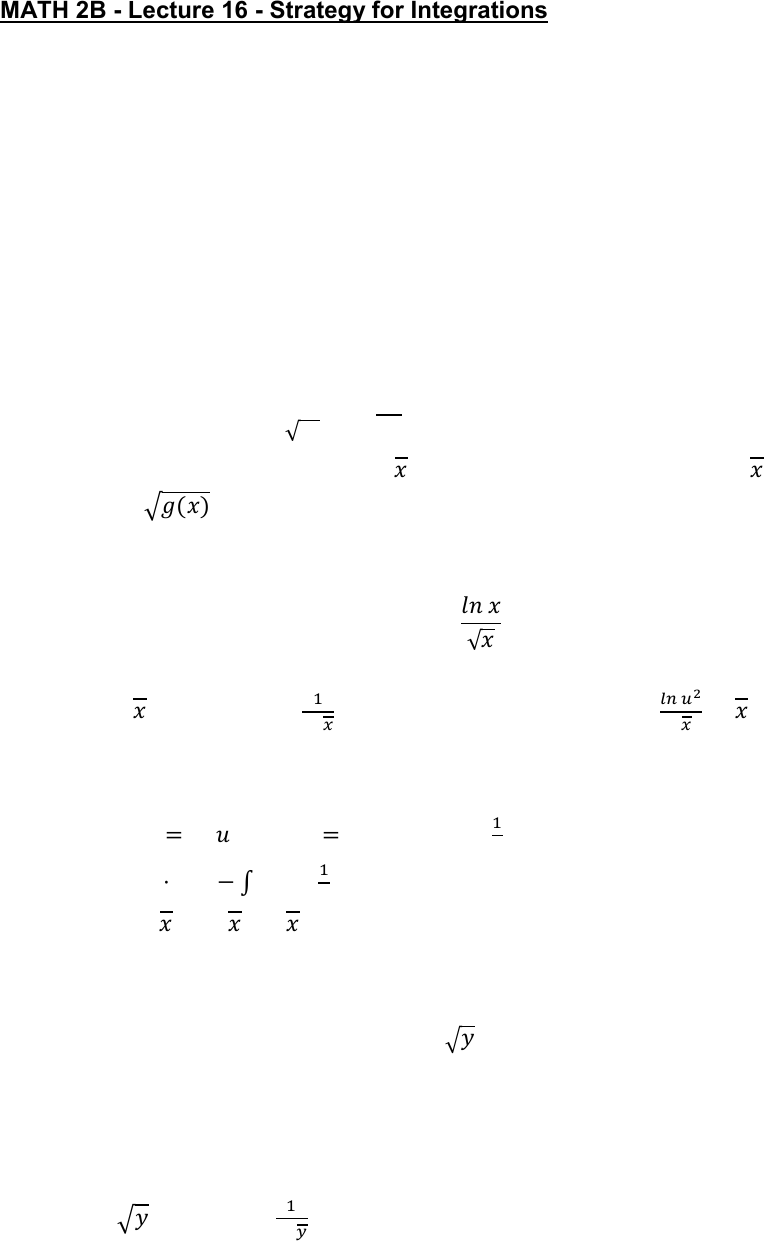Class Notes (1,100,000)
US (490,000)
UC-Irvine (20,000)
MATH (1,000)
MATH 2B (600)
Lecture 16

# MATH 2B Lecture Notes - Lecture 16: Trigonometric Substitution, Partial Fraction DecompositionPremium

Department
Mathematics
Course Code
MATH 2B
Professor
ERJAEE, G.
Lecture
16

This preview shows half of the first page. to view the full 3 pages of the document.02/11/2019
MATH 2B - Lecture 16 - Strategy for Integrations
In general, the process of integration is not very straightforward. It usually requires more
than one method to solve for one integral.
List of strategies:
1. u-substitution
2. Integration-by-parts
3. Trigonometric integrals
4. Trigonometric substitution
5. Integration by partial fraction
Since u-sub is the most basic one. It is very usual that we will need u-sub
together with another one of the strategies
If we observe there is in the integral, we can try . If there is
, we can try   
Example:


Solution:
 
 

   
Integration-by-parts:
    
  

   

Example:

Solution:
 
